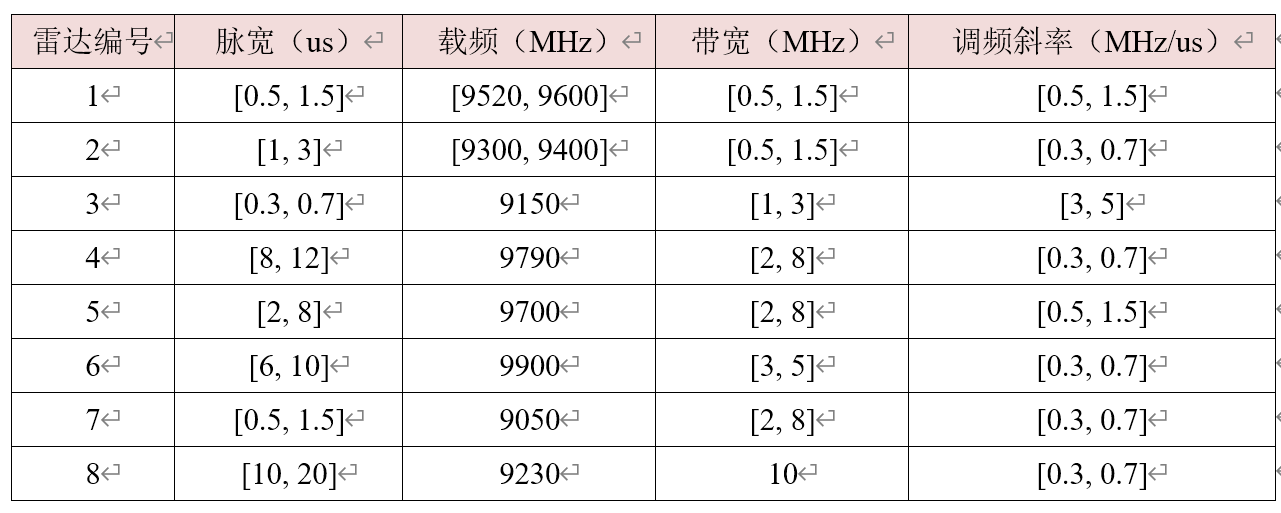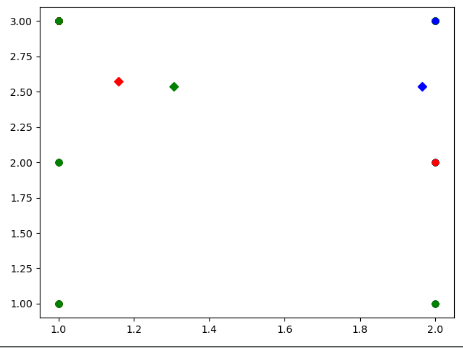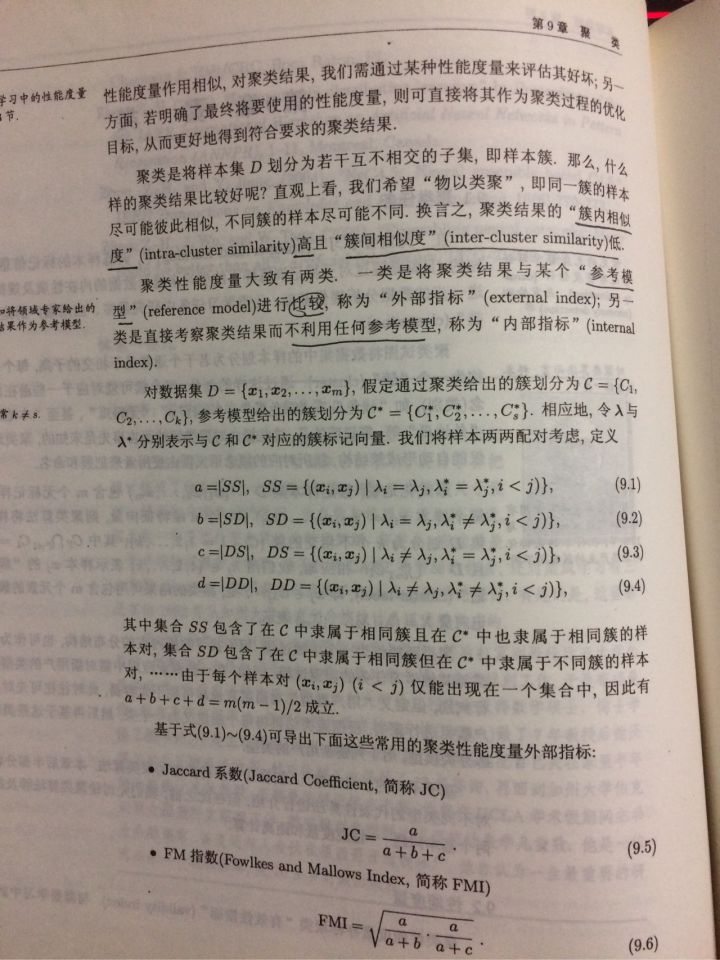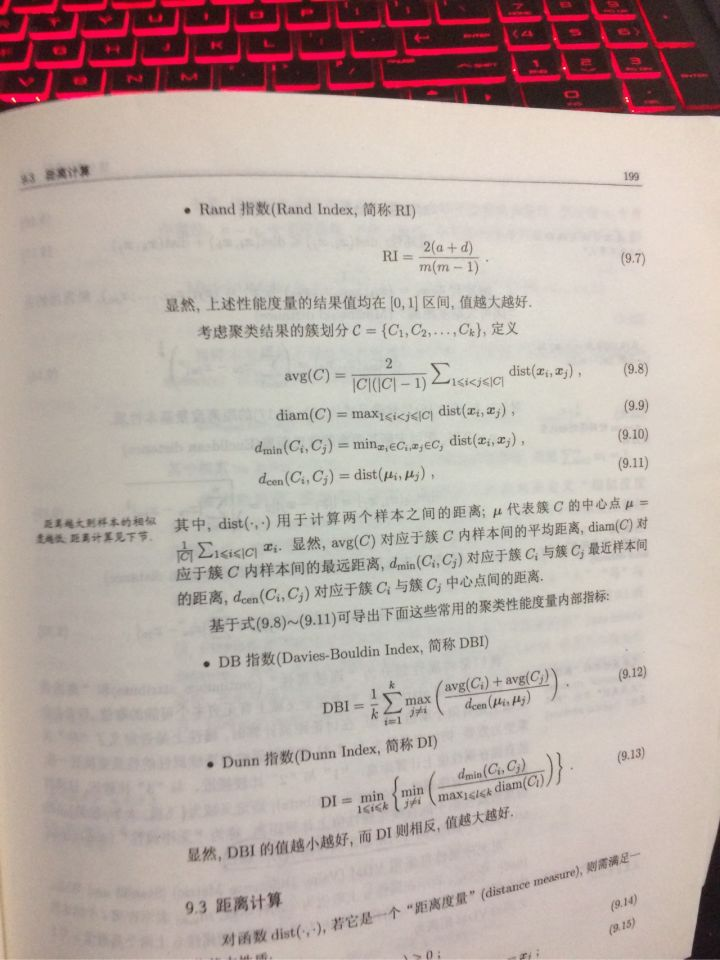• 多维数据聚类
千次阅读
2021-01-27 04:00:28

我一直试图用热图来实现k-means聚类，但是没有成功。在

以下是初始数据集：

我的密码是：import matplotlib.pyplot as plt

import numpy as np

import pandas as pd

import math

import random

#%matplotlib inline

def truncate(f, n):

return math.floor(f * 10 ** n) / 10 ** n

def chooseCenter(data, centers):

length = data.shape

cent = []

while len(cent) < centers :

x = random.randrange(0,length)

y = random.randrange(0,length)

if data.iloc[x][y] not in cent:

d = truncate(data.iloc[x][y],2)

cent.append(d)

return cent

def distance(val, center):

return math.sqrt((val- center)**2)

def getDistances(centers, data):

length = data.shape

dist = []

for i in range(length):

for j in range(length):

y = []

for k in range(len(centers)):

val = distance(data.iloc[i][j], centers[k])

y.append(truncate(val,3))

dist.append(y)

return dist

def findClosest(data, dist):

close = data.copy()

length = close.shape

indexes = []

for i in range(len(dist)):

pt = min(dist[i])

idx = dist[i].index(pt)

indexes.append(idx)

#print(indexes)

length = data.shape

n = np.array(indexes)

n = pd.DataFrame(np.reshape(n, (length,length)))

#reshape this data frame into the same shape as the data

#keep running the find closest until there is no change

#try heatmap on this?

#this should cluster it, but to make sure test it

#might need to do some tweaking to this

return n

# for i in range(length):

# for j in range(length):

# print('dist[i]', dist[j])

# pt = min(dist[j])

# print(pt)

# idx = dist[j].index(pt)

# close.iloc[i][j] = int(idx)

#return close

def computeNewCenter(data, close):

d = dict()

for i in range(len(close)):

for j in range(len(close)):

d[close.iloc[i][j]] = []

for i in range(len(data)):

for j in range(len(data)):

if close.iloc[i][j] in d:

d[close.iloc[i][j]].append(data.iloc[i][j])

newCenters = []

for key, value in d.items():

m = np.mean(value)

newCenters.append(truncate(m, 3))

return newCenters

# lst = [[] * numcenters]

# for i in range(len(close)):

# for j in range(len(close)):

# if close.iloc[i][j]

def main():

data = data.T

#print(data)

df = pd.DataFrame(data[1:], columns=data, dtype=float).T

df = df.iloc[::-1]

# print(df)

# print(df.iloc)

# print(df)

# print(df.iloc)

# heatmap = plt.pcolor(df, cmap=plt.cm.bwr)

# plt.colorbar(heatmap)

c = chooseCenter(df, 3)

print(c)

#print(len(c))

dist = getDistances(c, df)

#print(dist)

y = findClosest(df, dist)

# q = []

# for i in range(len(c)):

# q.append([])

# #print(q)

j = computeNewCenter(df, y)

#print(j)

length = df.shape

oldFrame = pd.DataFrame(np.ndarray((length,length)))

oldFrame = oldFrame.fillna(0)

ct=0

while y.equals(oldFrame) == False:

ct+=1

oldFrame = y.copy()

c = computeNewCenter(df, oldFrame)

#print(c)

dist = getDistances(c, df)

#print(dist)

y = findClosest(df, dist)

#print(y)

#plt.pcolor(df, cmap=plt.cm.bwr)

l = []

for i in range(len(y)):

for j in range(len(y)):

if y.iloc[i][j] == 1:

l.append(df.iloc[i][j])

for i in range(len(y)):

for j in range(len(y)):

if y.iloc[i][j] == 2:

l.append(df.iloc[i][j])

for i in range(len(y)):

for j in range(len(y)):

if y.iloc[i][j] == 0:

l.append(df.iloc[i][j])

l = np.ndarray((length,length))

l = pd.DataFrame(l)

print(l)

hm = plt.pcolor(l, cmap=plt.cm.bwr)

plt.colorbar(hm)

# print(y)

# print(c)

# print(ct)

#plt.pcolor(y, cmap=plt.cm.bwr)

if __name__ == '__main__':

main()

我的思路是：

我目前的想法是先随机选择中心。

然后为每个点到每个中心的距离创建一个列表。

求每个中心点的最小距离的指数。

创建一个与数据集大小相同的数据帧，并用点最接近的中心的索引填充每个元素的索引。

取中心指数相同的点的平均值重新计算中心

重复此过程多次，直到索引数据帧不变。

创建一个新的数据帧，并添加在数据框中具有相同中心点的点。

然后创建热图。在

不过，这似乎不起作用。

只是想知道，我是在正确的轨道上还是完全偏离了轨道，如果我在正确的轨道上，我需要改变哪些部分来解决问题。如果不能，请你给我指一下正确的路线。在

以下是地图对比：

第一个是我的程序生成的，第二个是它应该看起来的样子。在

我知道我的问题出在k-means聚类算法的某个部分，我的猜测是，要么是在重新分配阶段，即重新分配点到质心并计算新的质心，要么是处于停止状态，因为算法运行的时间不够长。同样在我的脑后，一些东西告诉我，我做这件事的效率并没有达到我所能达到的水平，而且我遗漏了一些关键的东西。我看了几段关于K-means聚类的视频，并从概念上理解了它，我只是很难实现它。在

更多相关内容
• 为了解决传统聚类方法在多维数据集中聚类效果不佳的问题，提出将网络社团划分的方法应用到多维数据聚类分析中。对于一个多维数据集，首先对分析对象进行特征提取，构建出每个对象的特征向量，通过计算皮尔森相关系数...
• 给定一组数据点，我们可以使用聚类算法将每个数据点划分为一个特定的组。理论上，同一组中的数据点应该具有相似的属性和/或特征，而不同组中的数据点应该具有高度不同的属性和/或特征。聚类是一种无监督学习的方法，...

# 聚类模型

聚类是一种机器学习技术，它涉及到数据点的分组。给定一组数据点，我们可以使用聚类算法将每个数据点划分为一个特定的组。理论上，同一组中的数据点应该具有相似的属性和/或特征，而不同组中的数据点应该具有高度不同的属性和/或特征。聚类是一种无监督学习的方法，是许多领域中常用的统计数据分析技术。
在数据科学中，我们可以使用聚类分析从我们的数据中获得一些有价值的见解。

## 高斯混合模型GMM

高斯混合模型（Gaussian Mixture Model, GMM）：

用概率模型来刻画聚类原型（软聚类）。GMM可以看作是由K个单高斯模型组合而成的模型，即概率密度分布其中， ϕ ( x ∣ θ k ) \mathop{\phi}(x|{\theta}_k) 为第k个单维高斯分布子模型的概率密度函数，参数为 θ k = ( μ k , σ k 2 , α k ) \mathop{\theta}_k=({\mu}_k,{\sigma}^2_k,{\alpha}_k) ，分别表示每个子模型的期望、方差和混合系数。混合系数 α k \mathop{\alpha}_k 指的是子模型k在混合模型中发生的概率。

针对GMM的聚类问题，我们采用极大似然估计方法（Maximum Likelihood Estimation, MLE），求解每个子模型的参数 { θ k = ( μ k , σ k 2 , α k ) ∣ k ∈ [ 1 , K ] } \mathop\lbrace{\theta}_k=({\mu}_k,{\sigma}^2_k,{\alpha}_k)|k\in[1,K]\rbrace 。对于单高斯模型，若每个数据点（个数N）是相互独立的，其对数似然函数为
log ⁡ L ( θ ) = ∑ j = 1 N log ⁡ ϕ ( x j ∣ θ ) . \begin{aligned} \log L({\theta}) = {\sum}_{j=1}^{N}\log \phi(x_j|\theta). \end{aligned}
对于GMM，其对数似然函数为
log ⁡ L ( θ ) = ∑ j = 1 N ∑ k = 1 K α k ϕ ( x ∣ θ k ) . \begin{aligned} \log L({\theta}) = {\sum}_{j=1}^{N}{\sum}_{k=1}^K\alpha_k\phi(x|\theta_k). \end{aligned}
对于上述求似然函数最大的问题，由于每个子分布是未知的，即隐遍历，我们常用EM算法(Expectation-Maximization)求解各个参数。EM算法的每次迭代包含两个步骤，即E步和M步：
① E步：
根据当前参数计算每个样本j来自子模型k的后验概率其中， j = 1 , 2 , … , N , k = 1 , 2 , … , K \mathop j=1,2,…,N,k=1,2,…,K
② M步：
{ θ k = ( μ k , σ k 2 , α k ) ∣ k ∈ [ 1 , K ] } \mathop\lbrace{\theta}_k=({\mu}_k,{\sigma}^2_k,{\alpha}_k)|k\in[1,K]\rbrace 能使似然函数最大，则分别对三个函数求偏导更新参数：其中， k = 1 , 2 , … , K \mathop k=1,2,…,K 。当停止条件满足时，停止迭代。

## 谱聚类

谱聚类（spectral clustering），是一种基于图论的聚类方法。。它的主要思想是把所有的数据看做空间中的点，这些点之间可以用边连接起来。距离较远的两个点之间的边权重值较低，而距离较近的两个点之间的边权重值较高，通过对所有数据点组成的图进行切图，让切图后不同的子图间边权重和尽可能的低，而子图内的边权重和尽可能的高，从而达到聚类的目的。

因此，概括来说，谱聚类的步骤主要有两个，一是构图，二是切图。构图时，样本对应的是图的顶点，样本相似度是边的权重。切图后的结果是同子图内顶点相似度高，不同子图内顶点相似度低。

谱聚类的主要步骤 ：

• 根据样本构建权重矩阵 W。
• 计算度矩阵 D 和拉普拉斯矩阵 L，对 L 进行奇异值分解（SVD）；
• 取 L 的前 K 个最小特征值对应的右奇异向量，构成矩阵 V。这一步的目的是给拉普拉斯特征映射降维度。
• 对 V 的行向量进行K-Means聚类。即对样本数据的拉普拉斯矩阵的奇异值向量进行聚类。

## 密度聚类DBSCAN

DBSCAN（Density-Based Spatial Clustering of Applications with Noise）是一种基于一组“邻域”参数来刻画样本分布的紧密程度的密度聚类方式。它是从样本密度的角度考察样本之间的可连接性，将簇定义为密度相连的点的最大集合，能够把具有足够高密度的区域划分为簇，并可在噪声的空间数据库中发现任意形状的聚类。

DBSCAN划分簇的方法为：若 x \mathop x 为核心对象，由 x \mathop x 密度可达的所有样本组成的集合为X，则 X 即为满足连接性与最大性的簇。其中，密度可达表示：

x j \mathop x_j 位于 x i \mathop x_i ϵ \mathop \epsilon - 邻域中，且 x i \mathop x_i 是核心对象，那么称 x j \mathop x_j x i \mathop x_i 密度直达。那么，若有一连串这样的 ( x n , x n − 1 , . . . , x 0 ) \mathop (x_n,x_{n-1},...,x_0) ，其中， x i \mathop x_i x i − 1 \mathop x_{i-1} 密度直达，那么 x n \mathop x_n x 0 \mathop x_0 密度可达。

密度可达关系如下图所示。# 聚类效果的指标

1.精度
精度（accuracy）表示聚类后，分选正确的样本数占样本总数的比例。
2.聚类纯度
聚类纯度（Purity），利用聚类后的每个簇中，占比最高的真实类别的元素个数计算。由于对于聚类后的结果我们并不知道每个簇所对应的真实类别，因此需要取每种情况下的最大值。具体的，纯度的计算公式定义如下：其中， N \mathop N 表示总的样本数。 Ω = { ω 1 , ω 2 , . . . , ω K } \mathop \Omega = \lbrace \omega_1,\omega_2,...,\omega_K\rbrace 表示聚类后的簇和集合， C = { c 1 , c 2 , . . . , c J } \mathop C = \lbrace c_1,c_2,...,c_J\rbrace 表示正确的类别。 ω k \mathop \omega_k 表示聚类后第 k \mathop k 个簇中所有的样本，而 c j \mathop c_j 表示第 j \mathop j 个类真实的样本。

例：该聚类的纯度为：(5+4+3)/17 =0.706。

3.混淆矩阵
混淆矩阵可以用于评估分类的准确性。根据定义，混淆矩阵如下灰色区域所示，其中 C i j \mathop C_{ij} 等于已知在簇i中，被预测为簇 j \mathop j 中的样本数量。# 实例：雷达信号分选

即，利用上述三种聚类方法分类雷达信号辐射源。

## 内容

假设环境中包含 8 部雷达，每部雷达的数据参数如下：对给出的 8 部雷达数据实现基于无监督聚类的信号分选，具体步骤为：

• 针对每部雷达，在其各参数区间内随机产生 500 个样本数据，每个样本包含上述四种特征数据（脉宽、载频、带宽和调频斜率）；
• 分别用 GMM、谱聚类、DBSCAN 三种算法进行聚类分选；
• 列表比较各算法的以下指标：精度、聚类纯度、辐射源级的查准率和查全率；
• 列表比较各算法的效率。

补充：辐射源级的查准率和查全率
对于信号分选，有时我们只关心是否能将电磁环境中存在的各个辐射源分选出来，也就是考察“辐射源级”的信号分选结果，而不会关注到“样本级”的粒度。
对于单个辐射源级的查准率（Precision）和查全率（Recall）定义如下：

① 查准率（Precision）：分选出的信息中我们关注的辐射源的信号的比例。
② 查全率（Recall）：我们关注的辐射源信号有多少比例被分选出来了。

## 代码

代码如下：
1.生成数据

import openpyxl
import numpy as np
import xlrd
import random
''' 函数2 产生的样本数据写入excel表格'''
def write_to_excel(value,sheet_name,filename):
# 雷达数据i = [脉宽,载频,带宽,调频斜率]*500
sheet = wb[sheet_name]
for item in value:
sheet.append(item)
wb.save(filename)
print('成功！')

''' 函数1 由雷达参数产生随机数据 '''
def sample_data(radar_para, N, Num):
[Tl, Tu, fcl, fcu, Bl, Bu, Kl, Ku] = radar_para
sample = []
for i in range(N):
T = np.random.uniform(Tl,Tu)
fc = np.random.uniform(fcl,fcu)
B = np.random.uniform(Bl,Bu)
K = np.random.uniform(Kl,Ku)
sample.append([T,fc,B,K,Num])  # Num是标签，无监督时用不上
return sample

# 读取雷达参数，也就是上文的那个表格
para_excel = xlrd.open_workbook('parameter.xlsx')
nRows = para_sheet.nrows     # 读取行数
nRadar = nRows -1
radar_para = []     # 雷达参数list
for i in range(nRows-1):
para = para_sheet.row_values(i+1)
print(para)
# 每个雷达的信号数据个数
N = 500

# 建立用于存放数据的excel表格
wb = openpyxl.Workbook()
sheet_name = 'data'
wb.create_sheet(sheet_name,0)
sheet = wb[sheet_name]
wb.save(filename)

# 向excel写数据
data = []
for i in range(nRadar):
data = data + shuju
write_to_excel(data,sheet_name,filename)     # 生成一个excel hxd！


2.聚类
DBSCAN为例.

准确率，辐射源级的查全率和查准率就不在这里写了。
这几个参数的计算需要进行标签映射：因为无监督聚类后，虽然本质上的同一类可能大部分都分到了一起，但是他的标签与原标签不一定是对应的。
标签映射需要设计一个简单的算法
这里可以说一下我个人的思路：看一下每个聚类得到的簇里面哪个真实类别最多，就把该类标记为哪个真实类别。

import time
import numpy as np
import pandas as pd
import matplotlib.pyplot as plt
from sklearn.cluster import DBSCAN
from sklearn import metrics
#----------------- Subfunction -------------------
def normalization(data):  # 数据归一化
_range = np.max(data) - np.min(data)
return (data - np.min(data)) / _range

begin_time = time.time() # 测试运行时间
# 自设颜色
colors = [] # 你喜欢的颜色~
[x, y] = radar_data_sheet.shape
# 对数据归一化处理 谱聚类不用归一化
#------------------- 模型 ------------------------
db = DBSCAN(eps=0.005, min_samples=10)  # DBSCAN
print(label_pred==-1)

# GMM
# gmm = GaussianMixture(
#    n_components = num_clusters, covariance_type="full", max_iter=20)
# label_pred = gmm.predict(radar_data_norm)  # 训练得到的label

# 谱聚类
#sp = SpectralClustering(n_clusters=num_clusters,
#                        assign_labels='discretize',
#                        random_state=0)
#label_pred = sp.fit_predict(X)   # 得到预测的 lable

#------------------- 聚类性能指标 ---------------------
print("簇数: %d" % num_clusters)
print("Completeness: %0.3f" % metrics.completeness_score(radar_label, label_pred))
print("Homogeneity: %0.3f" % metrics.homogeneity_score(radar_label, label_pred))
# 准确率还有查准率和查全率就不在这里写了

#---------------------- 绘图 -----------------------
plt.figure(1)
plt.figure(figsize=(10, 8))
# 原图
plt.subplot(2,2,1)
plt.title('Original data')
for i in range(num_clusters):
plt.scatter(data[:, 0],data[:, 1],s=2, color= colors[i])
plt.subplot(2,2,3)
plt.title('Original data')
for i in range(num_clusters):
plt.scatter(data[:, 2], data[:, 3], s=2, color=colors[i])

# 绘制聚类后的图
plt.subplot(2,2,2)
plt.title('DBSCAN')
for i in range(num_clusters):
data = radar_data[label_pred == i]
plt.scatter(data[:, 0],data[:, 1],s=2, color= colors[i])
plt.subplot(2,2,4)
plt.title('DBSCAN')
for i in range(num_clusters):
data = radar_data[label_pred == i]
plt.scatter(data[:, 2], data[:, 3], s=2, color=colors[i])

end_time = time.time()
run_time = end_time-begin_time
print ('DBSCAN 程序运行时间：',run_time)


## 结果簇数: 8
准确率: 100.00%
Completeness: 1.000
Homogeneity: 1.000
DBSCAN 程序运行时间： 1.3269813867223123

# 参考

 五种主要聚类算法
 sklearn官网

展开全文机器学习
• ## python实现K-means多维数据聚类代码

万次阅读 多人点赞 2020-06-10 17:03:20
python实现K-means多维数据聚类 #!/usr/bin/env python #-*- coding:utf-8 -*- # author:wanglubao # datetime:2019/9/22 14:31 # software: PyCharm import numpy as np import matplotlib.pyplot as plt import ...

python实现K-means多维数据聚类

#!/usr/bin/env python
#-*- coding:utf-8 -*-
# author:wanglubao
# datetime:2019/9/22 14:31
# software: PyCharm
import numpy as np
import matplotlib.pyplot as plt
import pandas as pd
#加载数据
#data = np.loadtxt(fileName, delimiter='\t', dtype=float, skiprows=1)
return data

#欧氏距离计算
def distEclud(x,y):
return np.sqrt(np.sum((x-y)**2))
# 为给定数据集构建一个包含K个随机质心的集合
def randCent(dataSet,k):
# 获取样本数与特征值
m,n = dataSet.shape#把数据集的行数和列数赋值给m,n
# 初始化质心,创建(k,n)个以零填充的矩阵
centroids = np.zeros((k,n))
# 循环遍历特征值
for i in range(k):
index = int(np.random.uniform(0,m))
# 计算每一列的质心,并将值赋给centroids
centroids[i,:] = dataSet[index,:]
# 返回质心
return centroids

# k均值聚类
def KMeans(dataSet,k):
m = np.shape(dataSet)
# 初始化一个矩阵来存储每个点的簇分配结果
# clusterAssment包含两个列:一列记录簇索引值,第二列存储误差(误差是指当前点到簇质心的距离,后面会使用该误差来评价聚类的效果)
clusterAssment = np.mat(np.zeros((m,2)))
clusterChange = True

# 创建质心,随机K个质心
centroids = randCent(dataSet,k)
# 初始化标志变量,用于判断迭代是否继续,如果True,则继续迭代
while clusterChange:
clusterChange = False

#遍历所有样本（行数）
for i in range(m):
minDist = 100000.0
minIndex = -1
# 遍历所有数据找到距离每个点最近的质心,
# 可以通过对每个点遍历所有质心并计算点到每个质心的距离来完成
for j in range(k):
# 计算数据点到质心的距离
# 计算距离是使用distMeas参数给出的距离公式,默认距离函数是distEclud
distance = distEclud(centroids[j,:],dataSet[i,:])
# 如果距离比minDist(最小距离)还小,更新minDist(最小距离)和最小质心的index(索引)
if distance < minDist:
minDist = distance
minIndex = j
# 如果任一点的簇分配结果发生改变,则更新clusterChanged标志
if clusterAssment[i,0] != minIndex:
clusterChange = True
# 更新簇分配结果为最小质心的index(索引),minDist(最小距离)
clusterAssment[i,:] = minIndex,minDist
# 遍历所有质心并更新它们的取值
for j in range(k):
# 通过数据过滤来获得给定簇的所有点
pointsInCluster = dataSet[np.nonzero(clusterAssment[:,0].A == j)]
# 计算所有点的均值,axis=0表示沿矩阵的列方向进行均值计算
centroids[j,:] = np.mean(pointsInCluster,axis=0)
print("Congratulation,cluster complete!")
# 返回所有的类质心与点分配结果
return centroids,clusterAssment

def showCluster(dataSet,k,centroids,clusterAssment):
m,n = dataSet.shape
#if n != 2:
# print("数据不是二维的")
#return 1

mark = ['or','ob','og','ok','^r','+r','sr','dr','<r','pr']
if k > len(mark):
print("k值太大了")
return 1
#绘制所有样本
for i in range(m):
markIndex = int(clusterAssment[i,0])
plt.plot(dataSet[i,0],dataSet[i,1],mark[markIndex])

mark = ['Dr', 'Db', 'Dg', 'Dk', '^b', '+b', 'sb', 'db', '<b', 'pb']
#绘制质心
for i in range(k):
plt.plot(centroids[i,0],centroids[i,1],mark[i])

plt.show()
dataSet = loadDataSet("C:/Users/Mr zhu/Desktop/cc.txt")
k = 3
centroids,clusterAssment = KMeans(dataSet,k)
showCluster(dataSet,k,centroids,clusterAssment)



数据图片：结果截图：展开全文机器学习
• 计算机研究 -基于粗糙集的多维数据聚类分析.pdf
• 计算机研究 -基于Global K-means的多维数据聚类算法研究及其GPU加速.pdf
• 聚类分析，模糊集，适用于多维数据聚类。在研究生期间所做的成功，成功将三位数据实现聚类，并把它运用到交通分类当中。-Cluster analysis, fuzzy sets, is applicable to multi-dimensional data clustering.During...
• 基于多维特征聚类和用户评分的景点推荐算法.pdf
• 一个刚编出来的K—means 聚类算法的matlab源代码 适合多维数据
• k-means高维聚类： https://www.cnblogs.com/icydengyw/p/13591990.html

python数据分析与挖掘 — 代码修正与项目练习：

https://blog.csdn.net/O_tongwandou/article/details/81952264

k-means高维聚类：

https://www.cnblogs.com/icydengyw/p/13591990.html

python代码实现TSNE降维数据可视化教程：

https://www.jb51.net/article/181578.htm

sklearn中的降维PCA与TSNE：

https://blog.csdn.net/qq_27584277/article/details/80770047

同为降维工具，二者的主要区别在于，

所在的包不同（也即机制和原理不同）

from sklearn.decomposition import PCA
from sklearn.manifold import TSNE


因为原理不同，导致，tsne 保留下的属性信息，更具代表性，也即最能体现样本间的差异；
TSNE 运行极慢，PCA 则相对较快；
因此更为一般的处理，尤其在展示（可视化）高维数据时，常常先用 PCA 进行降维，再使用 tsne：

data_pca = PCA(n_components=50).fit_transform(data)
data_pca_tsne = TSNE(n_components=2).fit_transform(data_pca)

转载https://blog.csdn.net/lanchunhui/article/details/64923702


几种常见的聚类评价指标：

https://zhuanlan.zhihu.com/p/343667804

聚类效果好坏的评价指标（附代码）：

https://zhuanlan.zhihu.com/p/57342770

聚类 | 超详细的性能度量和相似度方法总结：

https://blog.csdn.net/fengdu78/article/details/103951665

聚类︱python实现 六大 分群质量评估指标（兰德系数、互信息、轮廓系数）:

https://blog.csdn.net/sinat_26917383/article/details/70577710

聚类结果不好怎么办_人脸聚类常用评价指标：

https://blog.csdn.net/weixin_39628271/article/details/111007803

周志华西瓜书提到对聚类性能的指标：
外部指标和内部指标展开全文• 多维密度聚类的精细化道路交通运行状况检测.pdf
• DBSCAN 是一种非常著名的基于密度的聚类算法。其英文全称是 Density-Based Spatial Clustering of Applications with Noise，意即：一种基于密度，对噪声鲁棒的空间聚类算法。直观效果上看，DBSCAN 算法可以找到样本...
• 1、用户界面1)点击读取文件按钮，读取到的文件如下图所示：数据聚类系统读取文件数据聚类系统导入文件2)设置簇的个数，这里设置成2，并选择K-means聚类算法，显示的结果如下图：数据聚类系统运行K-means聚类算法3)...
• 该命令实现的是多维情况下的三维数据GMM聚类，该算法的缺点是使用matlab 对于大数据有计算机内存的要求。
• 首先导入必要的模块：import kmeansimport numpy as npimport matplotlib.pyplot as pltfrom math import sqrt(1) 从文件加载数据集构建数据矩阵，从文本中逐行读取数据，形成供后继使用的数据矩阵。dataSet=[]file...
• 在 如果您使用的是numpy，您将拥有一个数组： ^{pr2}$你也可能在处理一个列表 ^{3}$ 需要转换为array:np.array(X)，甚至是Pandas数据帧： 您可以通过执行以下操作来检查Pandas数据帧中的列类型：import pandas as ...
• 我的数据是115*64维的，需要进行聚类操作，于是自己动手进行了实验，运用的是matlab语言，最后选取部分进行可视化展示，效果还不错。（代码注释完整）
• https://blog.csdn.net/u013946150/article/details/112257043 https://blog.csdn.net/u013946150/article/details/112257063 https://blog.csdn.net/u013946150/article/details/112257075 ...https://blog.csdn.ne
• K均值算法，将数据矩阵命名为data，设置聚类簇个数k，可对多维数据进行聚类
• #多维特征数据进行聚类分析 from sklearn.pipeline import make_pipeline from sklearn.preprocessing import StandardScaler from sklearn.cluster import KMeans import pandas as pd #导入数据 df=pd....
• 昨天实现推送了，GMM高斯混合的EM算法实现的完整代码，这是不掉包的实现，并且将结果和sklearn中的掉包实现做了比较：聚类结果基本一致，要想了解这个算法实现代码的小伙伴，可以参考： 机器学习高斯混合...
• Dbscan聚类源代码。 可以实现对多维数据进行聚类
• 聚类算法直接从数据的内在性质中学习最优的划分结果或者确定离散标签类型。虽然在 Scikit-Learn 或其他地方有许多聚类算法，但最简单、最容易理解的聚类算法可能还得算是 k-means 聚类算法了，在sklearn.cluster....
• ## 高维数据聚类方法

万次阅读 多人点赞 2019-08-05 21:18:02
1一般数据聚类方法 聚类是一个无监督的分类,它没有任何先验知识可用. 典型的聚类过程主要包括数据(或称之为样本或模式)准备、特征选择和特征提取、接近度计算、聚类(或分组)、对聚类结果进行有效性评估等步骤. ...
• 一 、聚类 cluster.affinityPropagation 适合高维、多数据快速聚类 不需要指定最终聚类族的个数 对数据的初始值不敏感 ...然后使用少量实例样本作为聚类中心来描述数据集，聚类中心时数据集种最能代......